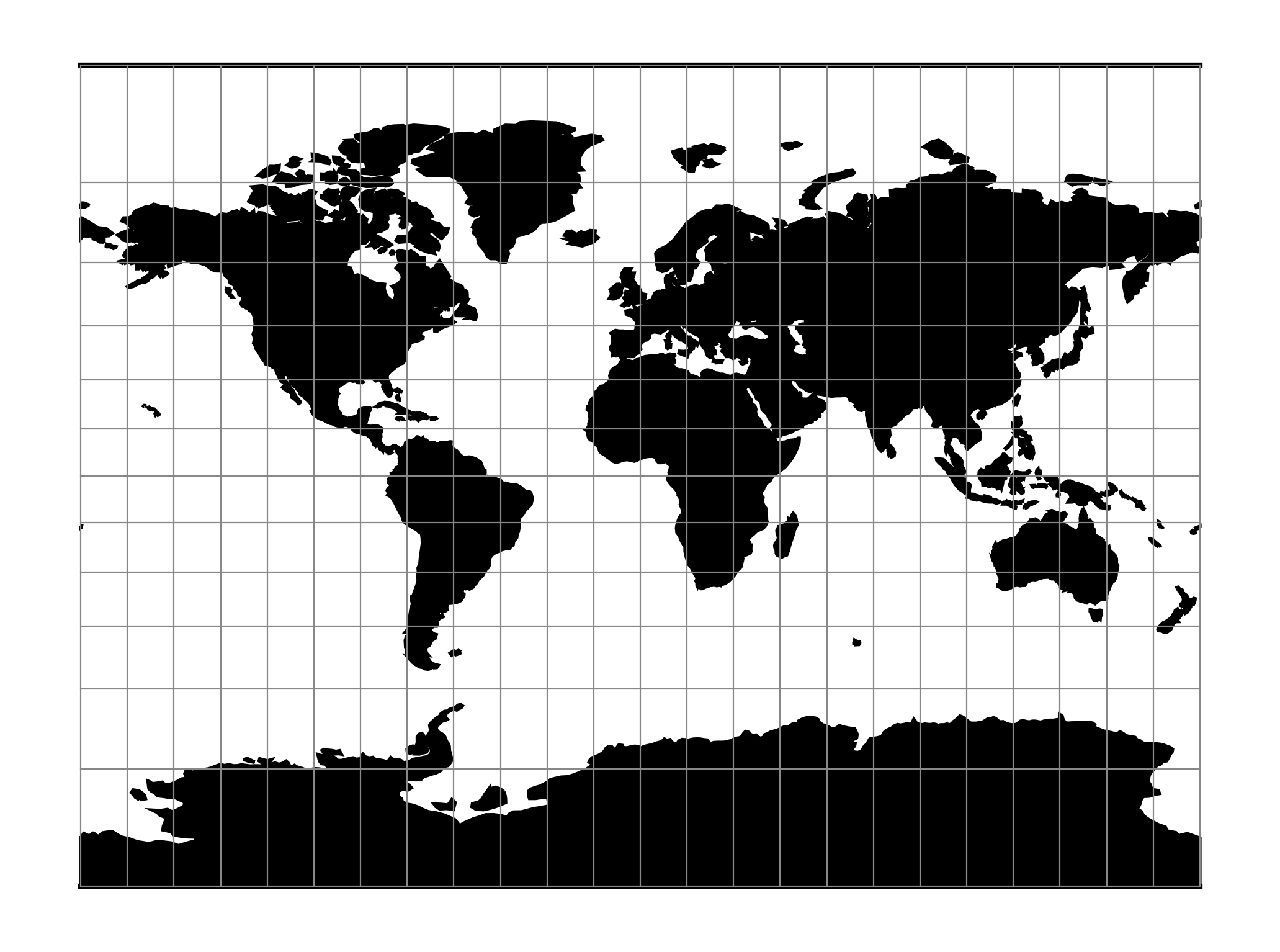# Miller Cylindrical¶

The Miller cylindrical projection is a modified Mercator projection, proposed by Osborn Maitland Miller in 1942. The latitude is scaled by a factor of $$\frac{4}{5}$$, projected according to Mercator, and then the result is multiplied by $$\frac{5}{4}$$ to retain scale along the equator.

 Classification Neither conformal nor equal area cylindrical Available forms Forward and inverse spherical Defined area Global, but best used near the equator Alias mill Domain 2D Input type Geodetic coordinates Output type Projected coordinatesproj-string: +proj=mill

## Usage¶

The Miller Cylindrical projection is used for world maps and in several atlases, including the National Atlas of the United States (USGS, 1970, p. 330-331) [Snyder1987].

Example using Central meridian 90°W:

\$ echo -100 35 | proj +proj=mill +lon_0=90w
-1113194.91      4061217.24


## Parameters¶

Note

All parameters for the projection are optional.

+lon_0=<value>

Longitude of projection center.

Defaults to 0.0.

+R=<value>

Radius of the sphere given in meters. If used in conjunction with +ellps +R takes precedence.

+x_0=<value>

False easting.

Defaults to 0.0.

+y_0=<value>

False northing.

Defaults to 0.0.

## Mathematical definition¶

The formulas describing the Miller projection are all taken from [Snyder1987].

### Forward projection¶

$x = \lambda$
$y = 1.25 * \ln \left[ \tan \left(\frac{\pi}{4} + 0.4 * \phi \right) \right]$

### Inverse projection¶

$\lambda = x$
$\phi = 2.5 * ( \arctan \left[ e^{0.8 * y} \right] - \frac{\pi}{4} )$